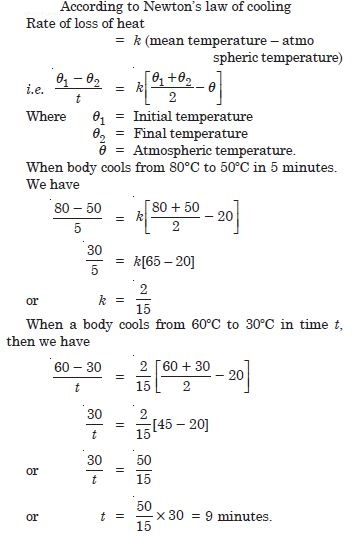# NCERT Solutions for Class 11 Physics Chapter 11 - Thermal Properties of Matter

##### Question 1:

The triple points of neon and carbon dioxide are 24·57 K and 216·55 K respectively. Express these temperatures on the Celsius and Fahrenheit scales.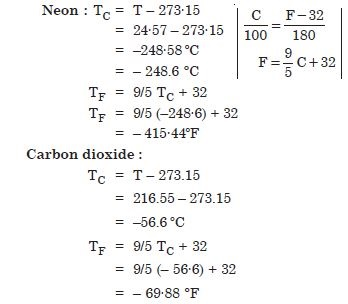##### Question 2:

Two absolute scales A and B have triple points of water defined to be 200 A and 350 B. What is the relation between TA and TB ?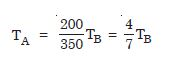##### Question 3: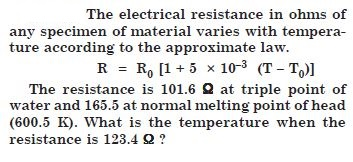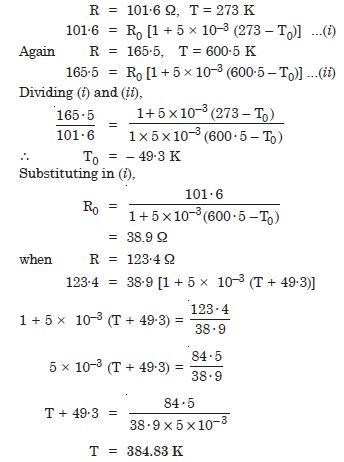##### Question 4:

The triple-point of water is a standard fixed point in modern thermometry, why ? What is wrong in taking the melting point of ice and the boiling point of water as standard point 1 as was originally done in the celsius scale ?

Triple point has a unique temperature. Fusion point and boiling point temperatures depend on pressure.

##### Question 5:

There were two fixed points in the original celsius scale as mentioned above which were assigned the number 0 $°$C and 100 $°$C respectively. On the absolute scale, one of the fixed points is the triple point of water which on the kelvin absolute scale is assigned the number 273.16 K. What is the other fixed point on this (kelvin) scale ?

The other fixed point is the absolute zero
i.e. (–273·16 $°$C)

##### Question 6:

The absolute temperature (kelvin scale) T is related to the temperature TC on the celsius scale by
TC = T – 273·15
Why do we have 273.15 in this relation and not 273·16 ?

Triple point is 0·01$°$C, not 0$°$C.

##### Question 7:

What is the temperature of the triple point of water on an absolute scale whose unit interval size is equal to that of the Fahrenheit scale ?

Temperature of triple point of water on absolute scale is given by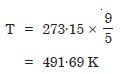##### Question 8:

Two ideal gas thermometers A and B use oxygen and hydrogen respectively. The following observations are made :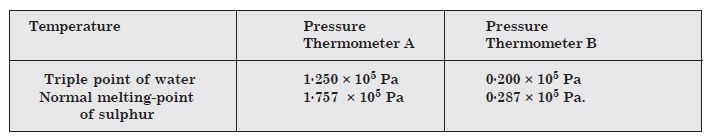What is the absolute temperature of normal melting point of sulphur as read by thermometers A and B ?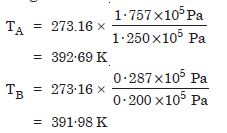##### Question 9:

Two ideal gas thermometers A and B use oxygen and hydrogen respectively. The following observations are made :What do you think to the reason for slightly different answers from A to B ? (The thermometers are not faulty).

The discrepancy arises because the gases are not perfectly ideal.

##### Question 10:

Two ideal gas thermometers A and B use oxygen and hydrogen respectively. The following observations are made :What further procedure is needed in the experiment to reduce the discrepancy between the two readings ?

To reduce the discrepancy, readings should be taken for lower pressures and the plot between temperature measured versus absolute of the gas at triple point should be extrapolated to obtain temperature in the limit pressure tends to zero, when the gases approach ideal gas behaviour.

##### Question 11:

A steel tape 1 metre long is correctly calibrated for temperature of 27·0$°$C. The length of a steel rod measured by this tape is found to be 63·0 cm on a hot day when the temperature is 45$°$C. What is the actual length of the steel rod on that day ? What is the length of the same steel rod on a day when the temperature is 27·0 $°$C ? Coefficient of linear expansion of steel = 1·20 × 10–5 C–1 :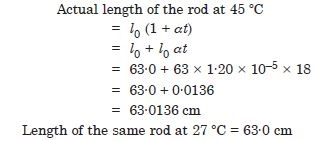##### Question 12:

A large steel wheel is to be fitted on a shaft of the same material. At 27 $°$C, the outer diameter of the shaft is 8·70 cm and the diameter of the central hole in the wheel is 8·69 cm. The shaft is cooled using ‘dry ice’. At what temperature of the shaft does the wheel slip on the shaft ? Assume coefficient of linear expansion of the steel is to be constant over the required temperature range : asteel = 1·20 × 10–5 K.

Original temperature = 27$°$C
Circumference of shaft = p × 8·7 cm
Circumference of wheel = p × 8·69 cm
In order that the steel wheel may slip over the shaft it should be heated till its circumference equals that of the shaft. Let t be temperature to which it must be heated, then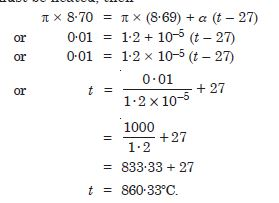##### Question 13:

A hole is drilled in a copper sheet. The diameter of the hole is 4·24 cm at 27 $°$C. What is the change in the diameter of the hole when the sheet is heated to 227$°$C ? Coefficient of linear expansion of copper = 1·70 × 105 $°$C–1.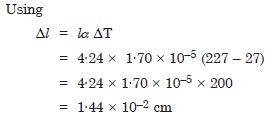##### Question 14:

A brass wire 1·8 m long at 27 $°$C is held when taut with little tension between two rigid supports. If the wire is cooled to a temperature – 39 $°$C, what is the tension developed in the wire, if its diameter is 2·0 mm ? Coefficient of linear expansion of brass = 2·0 × 10–5 $°$C–1, Young’s modulus of brass = 0·91 × 1011Pa.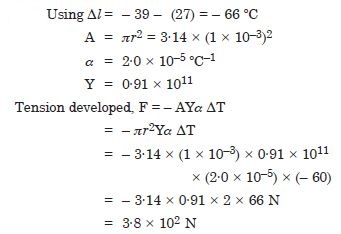##### Question 15:

A brass rod of length 50 cm and diameter 3·00 mm is joined to a steel rod of the same length and diameter. What is the change in length of the combined rod at 250 $°$C, if the original lengths are at 40·0 $°$C ? Is there a ‘thermal stress’ developed at the junction ? The ends of the rod are free to expand coefficient of linear expansion of brass = 2·0 × 10–5 $°$C–1, steel = 1·2 × 10–5 $°$C–1).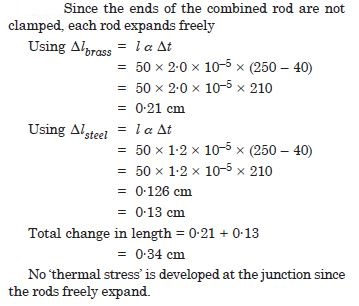##### Question 16:

The coefficient of volume expansion of glycerine is 49 × 10–5 $°$C–1. What is the fractional change in its density for 30 $°$C rise in temperature ?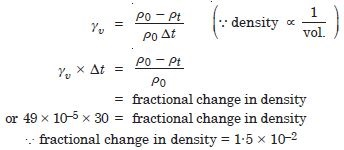##### Question 17:

A 10 kW drilling machine is used to drill a bore in a small aluminium block of mass 8.0 kg. How much is the rise in temperature of the block in 2.5 minutes, assuming 50% of power is used up in heating the machine itself or lost to the surroundings. Specific heat of aluminium = 0.91 J g–1 $°$C–1.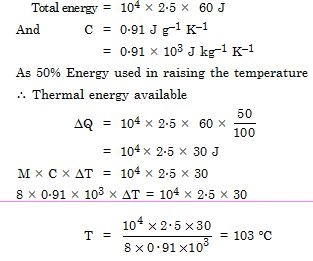##### Question 18:

A copper block of mass 2·5 kg is heated in a furnace to a temperature of 500 $°$C and then placed on a large ice block. What is the maximum amount of ice that can melt ? (Specific heat of copper = 0·39 J g–1 C–1, heat of fusion of water = 335 J g–1).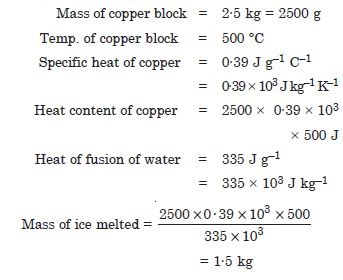##### Question 19:

In an experiment on the specific heat of a metal, a 0·20 kg block of the metal at 150 $°$C is dropped in a copper calorimeter (of water equivalent 0·025 kg) containing 150 cc of water at 27 $°$C. The final temperature is 40 $°$C. Compute the specific heat of the metal. If heat losses to the surroundings are not negligible, is your answer greater or smaller than the actual value for specific heat of the metal ?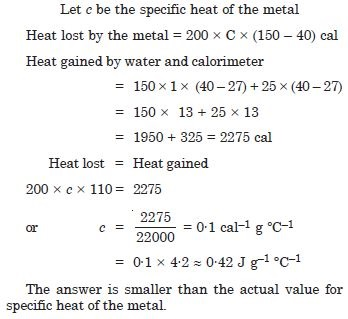##### Question 20:

Given below are observations on molar specific heats at room temperature of some common gases :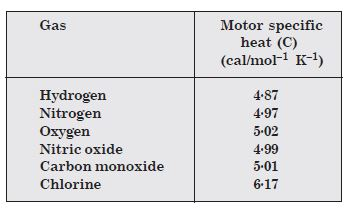The measured molar specific heats of these gases are markedly different from those for monoatomic gases.
(Typically, molar specific heat of a mono-atomic gas is 2.92 cal/mol K). Explain this difference. What can you infer from the somewhat larger (than the rest) value for chlorine ?

The gases are diatomic, and have other degrees of freedom (that is have other modes of motion) possible besides the translational degrees of freedom. To raise the temperature of the gas by a certain amount, heat is to be supplied to increase the average energy of all the modes. Consequently, molar specific heat of diatomic gases is more than that of monoatomic gases. It can be shown that if only rotational modes of motion are considered, the molar specific heat of diatomic gases is nearly 5/2. R which agrees with the observations for all the gases listed in the table except chlorine. The higher value of molar specific heat of chlorine indicates that besides rotational modes, vibrational modes are also present in chlorine at room temperature.

##### Question 21:

Answer the following question based on the P-T phase diagram of carbon dioxide :
At what temperature and pressure can the solid, liquid and vapour phases of CO2 co- exist in equilibrium ?

At the triple point temperature = – 56·6 $°$C and pressure = 5·11 atm.

##### Question 22:

Answer the following question based on the P-T phase diagram of carbon dioxide :
What is the effect of decrease of pressure on the fusion and boiling point of CO2 ?

Both the boiling point and freezing point of CO2 decrease if pressure decreases.

##### Question 23:

Answer the following question based on the P-T phase diagram of carbon dioxide :
What are the critical temperature and pressure for CO2 ? What is their significance ?

The critical temperature and pressure of CO2 are 31·1 $°$C and 73·0 atm respectively. Above this temperature, CO2 will not liquefy even if compressed to high pressures.

##### Question 24:

Answer the following question based on the P-T phase diagram of carbon dioxide :
In CO2 solid, liquid or gas (a) –70 $°$C under 1 atm. (b) –60 $°$C under 10 atm. (c) 15 $°$C under 56 atm.

(a) Vapour (b) Solid (c) Liquid.

##### Question 25:

Answer the following question based on the P–T phase diagram of CO2 (Fig. 11.1)
CO2 at 1 atm pressure and temperature – 60ºC is compressed isothermally. Does it go through a liquid phase ?

No, vapour condenses to solid directly.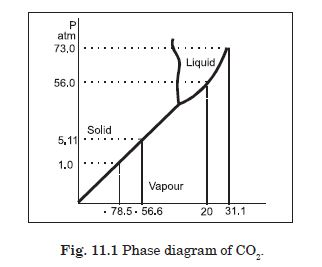##### Question 26:

Answer the following question based on the P–T phase diagram of CO2 (Fig. 11.1)
What happens when CO2 at 4 atm pressure is cooled from room temperature at constant pressure ?

It condenses to solid directly without passing through the liquid phase.##### Question 27:

Answer the following question based on the P–T phase diagram of CO2 (Fig. 11.1)
Describe qualitatively the changes in a given mass of solid CO2 at 10 atm pressure and temperature – 65ºC as it is heated upto room temperature at constant pressure.

It turns to liquid phase and then to vapour phase. The fusion and boiling points are when the horizontal line on P–T diagram at constant pressure of 10 atm intersects the fusion and vaporization curves.##### Question 28:

Answer the following question based on the P–T phase diagram of CO2 (Fig. 11.1)
CO2 is heated to a temperature 70ºC and compressed isothermally. What changes in its properties do we expect to observe ?

It will not exhibit any clear phase transition to liquid phase, but it will depart more and more from ideal gas behaviour as its pressure increases.##### Question 29:

A child running a temperature of 101 $°$F is given an antipyrin (i.e. a medicine that lowers fever) which causes an increase in the rate of evaporation of sweat from his body. If the fever is brought down to 98 $°$F in 20 min., what is the average rate of extra evaporation caused by the drug ? Assume the evaporation mechanism to be the only way by which heat is lost. The mass of the child is 30 kg. The specific heat of human body is approximately the same as that of water, and latent heat of evaporation of water at that temperature is about 580 cal g–1.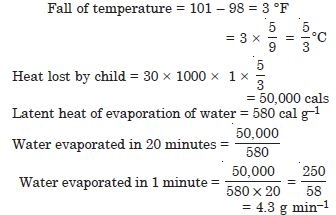##### Question 30:

A thermocole box is a cheap and efficient method for storing small quantity of cooked food in summer in particular. A cubical box of side 30 cm has a thickness of 5 cm. If 4 kg of ice are put in a box. Estimate the amount of ice remaining after 6 h. The outside temperature 45 ºC, and coefficient K = 0·01 J s–1m–1 K–1 [Heat of fusion of water = 335 × 103 J kg–1].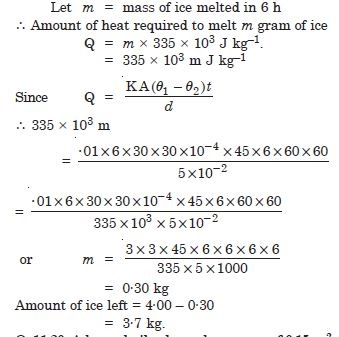##### Question 31:

A brass boiler has a base area of 0.15 m2 and thickness 1·0 cm. It boils water at the rate of 6·0 kg/min. when placed on a gas stove. Estimate the temperature of the part of the flame in contact with boiler. Thermal conductivity of brass = 109 J s–1 m–1 ºC–1;
Heat of vaporisation = 2256 × 103 J kg–1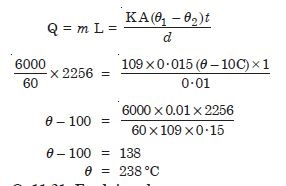##### Question 32:

Explain why :
A body with large reflecting is a poor emitter.

Good reflectors are poor absorbers and poor emitters.

##### Question 33:

Explain why :
A brass tumbler feels much colder than a wooden tray on a chilly day.

Because brass tumbler is good conductor of heat, on touching, heat quickly flows from our body to the tumbler and it appears colder. On the other hand, wood is a bad conductor of heat so on touching heat does not flow from our body to the wood and it appears comparatively hotter.

##### Question 34:

Explain why :
An optical pyrometer (for measuring high temperature) calibrated for an ideal black body radiation gives too low value for the temperature of a red hot iron piece in the open, but gives a correct value for the temperature when the same piece is in the furnace.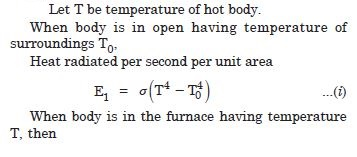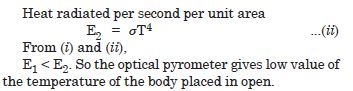##### Question 35:

Explain why :
The earth without its atmosphere would be in hospitably cold.

The temperature of earth is maintained by convection currents set in air which will not be possible with atmosphere.

##### Question 36:

Explain why :
Heating systems based on circulation of steam are more efficient in warming a building than those based on circulation of hot water.

A body cools from 80 ºC to 50 $°$C in 5 minutes. Calculate the time it takes to cool from 60 $°$C to 30 $°$C. The temperature of surrounding is 20 $°$C.